# Tetris randomizer

### Simple simulation of O(1) piece distribution calculation for a bag7-like Tetris randomizer

I wrote this tetris randomizer script a while ago and wanted to put it up here since there's some fun analysis to be done.

The gist of how it works is that every time a piece is picked, the chance that the chosen piece is picked again drops by a set amount, and that amount is distributed evenly over all the other pieces' weights. It's pretty simple but I had fun with it.

```import random
from matplotlib import pyplot as plt
import numpy as np
import pandas as pd
```
```PIECES = ['L', 'J', 'I', 'O', 'S', 'T', 'Z']
```
```# the heart of the randomizer
def alter_weights(current_weights, chosen_piece_index, denominator):
offset = (denominator - 1) * current_weights[chosen_piece_index] / denominator
updated_weights = [
weight + (offset / (len(PIECES) - 1)) if i != chosen_piece_index else weight - offset
for i,weight in enumerate(current_weights)
]
return updated_weights
```

As mentioned, this function is the heart of the randomizer. It chooses how to alter the piece weights based on the current weights and the chosen piece.

The denominator is very important here, as basically determines how much to alter the current weights at each iteration (effectively, a scaling parameter on weight volatility).

```# some more simple utilities

def choose_piece(weights):
return random.choices(range(len(PIECES)), weights=weights, k=1)

def copy(some_list):
return [i for i in some_list]
```
```# simulation logic
def simulate(N, denominator):
weights_over_time = []
pieces = []
weights =  * len(PIECES)
weights_over_time.append(copy(weights))
for _ in range(N):
next_piece = choose_piece(weights)
pieces.append(next_piece)
weights = alter_weights(weights, next_piece, denominator)
weights_over_time.append(copy(weights))
return weights_over_time, pieces
```

The logic for the simulation itself is pretty simple, starting with 1 for all weights and perturbing them over time.

```# plotting logic

def label(pair):
num, piece = pair
if type(piece) == int:
return str(num) + ':' + PIECES[piece]
return str(num) + ':' + piece

# see how frequent pieces are at each point in time
def stacked_barplot(weights_over_time, pieces, n, denominator, xticks=False):
x = [label(x) for x in zip(range(1, len(weights_over_time) + 1), ['(None)'] + pieces)]
y = []
for i in range(len(PIECES)):
y.append(
np.array(
[weights[i] for weights in weights_over_time]
)
)
for i in range(len(PIECES)):
plt.bar(x, y[i], bottom=sum(y[:i]), width=1.0)
plt.xlabel('Time')
plt.ylabel('Piece Weight Distribution')
if xticks:
plt.xticks(rotation='vertical', fontsize=4)
else:
plt.xticks([], [])
plt.title(f'Piece weight distribution over time ({n} iterations, denom = {denominator})')
plt.legend(PIECES)
plt.show()

# see how frequent pieces are on aggregate
def piece_frequency_histogram(pieces, n, denominator):
plt.hist(pieces, bins=len(PIECES), density=True, rwidth=0.5)
plt.title(f'Histogram of piece frequency ({n} iterations, denom = {denominator})')
plt.show()
```

With all the code out of the way, let's see how this performs.

We'll do a small simulation and a large simulation for each denominator value we test.

```# small simulation - denominator = 1.0
N = 100
DENOMINATOR = 1.0
sample_data, pieces = simulate(N, DENOMINATOR)

stacked_barplot(sample_data, pieces, N, DENOMINATOR)
piece_frequency_histogram(pieces, N, DENOMINATOR)
```
```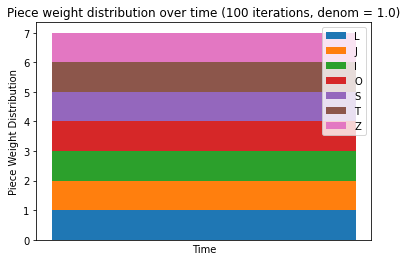```
```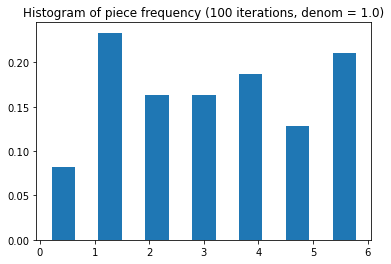```
```# large simulation - denominator = 1.0
N = 1000
DENOMINATOR = 1.0
sample_data, pieces = simulate(N, DENOMINATOR)

stacked_barplot(sample_data, pieces, N, DENOMINATOR)
piece_frequency_histogram(pieces, N, DENOMINATOR)
```
```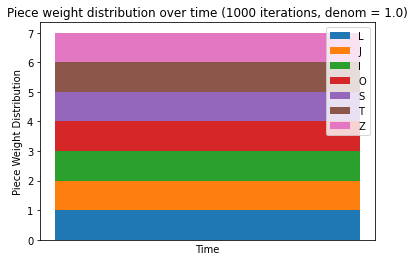```
```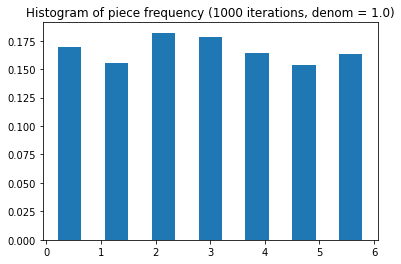```

As expected, with a denominator of 1, weights don't change at all throughout the process. But this doesn't mean piece weights don't change - in fact, looking at the piece frequencies over the small sample, it looks like they change quite a lot, with some pieces less than half as frequent as other pieces.

Part of the point of this randomizer is to minimize these drastic deviations in piece frequencies. Higher denominators will do a better job of this (as they actually change the piece distributions to counteract these deviations in piece frequency).

Now, just to get it out of the way, let's look at a small simulation with a denominator less than 1.

```# small simulation - denominator = 0.8
N = 100
DENOMINATOR = 0.8
sample_data, pieces = simulate(N, DENOMINATOR)

stacked_barplot(sample_data, pieces, N, DENOMINATOR)
piece_frequency_histogram(pieces, N, DENOMINATOR)
```
```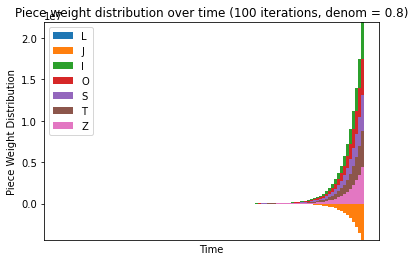```
```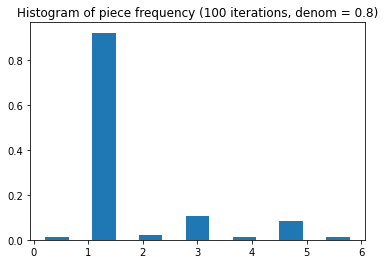```

With a denominator less than 1, the chosen piece actually has its weight increased, leading to an out-of-control upward trend for that piece and a trend towards 0 for everything else.

```# small simulation - denominator = 1.1
N = 100
DENOMINATOR = 1.1
sample_data, pieces = simulate(N, DENOMINATOR)

stacked_barplot(sample_data, pieces, N, DENOMINATOR)
piece_frequency_histogram(pieces, N, DENOMINATOR)
```
```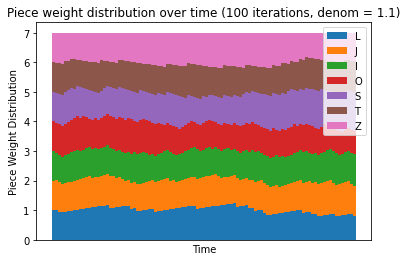```
```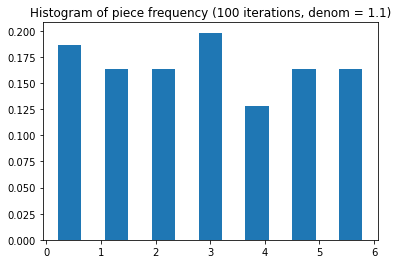```
```# large simulation - denominator = 1.1
N = 1000
DENOMINATOR = 1.1
sample_data, pieces = simulate(N, DENOMINATOR)

stacked_barplot(sample_data, pieces, N, DENOMINATOR)
piece_frequency_histogram(pieces, N, DENOMINATOR)
```
```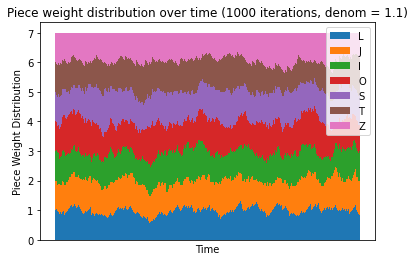```
```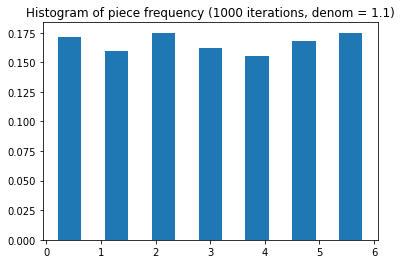```

Just this small increase in the denominator has increased piece weight volatility and stabilized piece frequencies significantly. Allowing piece weights to be affected by the previous frequencies has created an effect which prevents long-term domination by a single type, or several types, of pieces.

```# small simulation - denominator = 1.5
N = 100
DENOMINATOR = 1.5
sample_data, pieces = simulate(N, DENOMINATOR)

stacked_barplot(sample_data, pieces, N, DENOMINATOR)
piece_frequency_histogram(pieces, N, DENOMINATOR)
```
```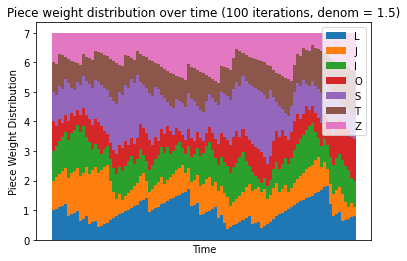```
```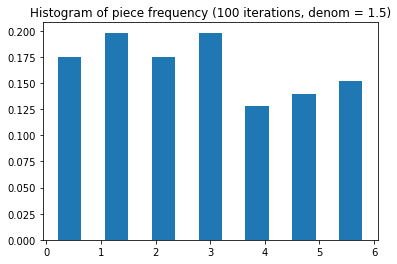```
```# large simulation - denominator = 1.5
N = 1000
DENOMINATOR = 1.5
sample_data, pieces = simulate(N, DENOMINATOR)

stacked_barplot(sample_data, pieces, N, DENOMINATOR)
piece_frequency_histogram(pieces, N, DENOMINATOR)
```
```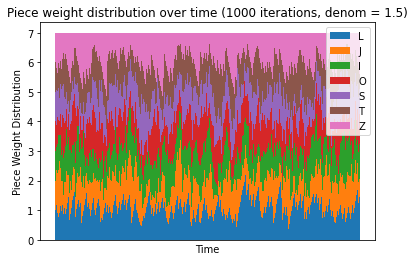```
```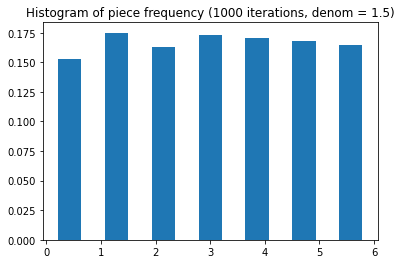```

Interestingly enough, the increase to 1.5 and subsequent sharp increase in weight volatility seems to have destabilized the piece frequencies again, with many pieces able to have their weights grow much more sharply over time rather than with the smoother increases as given by the denominator of 1.1.

Just to see what happens, let's try increasing the denominator a lot.

```# small simulation - denominator = 100
N = 100
DENOMINATOR = 100
sample_data, pieces = simulate(N, DENOMINATOR)

stacked_barplot(sample_data, pieces, N, DENOMINATOR)
piece_frequency_histogram(pieces, N, DENOMINATOR)
```
```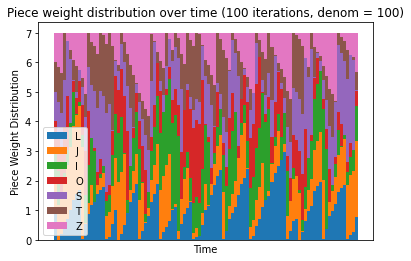```
```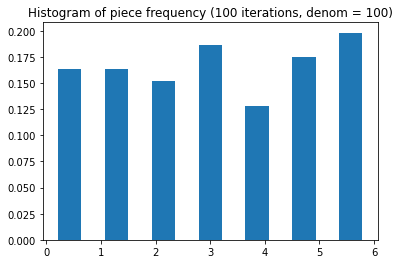```
```# large simulation - denominator = 100
N = 1000
DENOMINATOR = 100
sample_data, pieces = simulate(N, DENOMINATOR)

stacked_barplot(sample_data, pieces, N, DENOMINATOR)
piece_frequency_histogram(pieces, N, DENOMINATOR)
```
```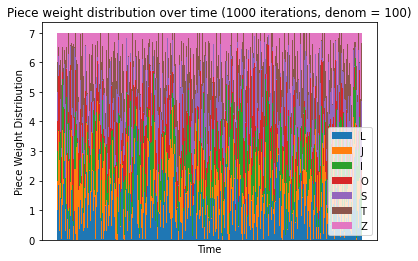```
```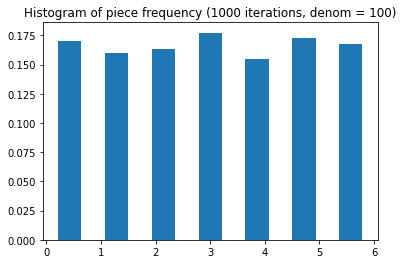```

This is pretty chaotic, but it looks like there's a limit to the instability that comes with a larger denominator. Shall we take it to its limit?

```# small simulation - denominator = 1E100
N = 100
DENOMINATOR = 1E100
sample_data, pieces = simulate(N, DENOMINATOR)

stacked_barplot(sample_data, pieces, N, DENOMINATOR)
piece_frequency_histogram(pieces, N, DENOMINATOR)
```
```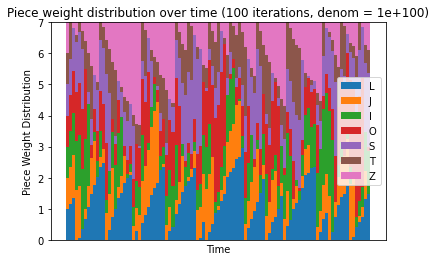```
```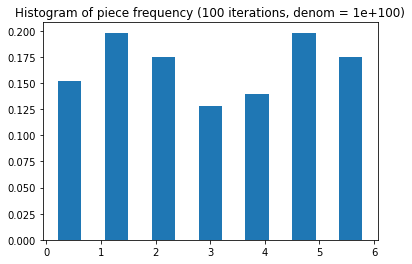```
```# large simulation - denominator = 1E100
N = 1000
DENOMINATOR = 1E100
sample_data, pieces = simulate(N, DENOMINATOR)

stacked_barplot(sample_data, pieces, N, DENOMINATOR)
piece_frequency_histogram(pieces, N, DENOMINATOR)
```
```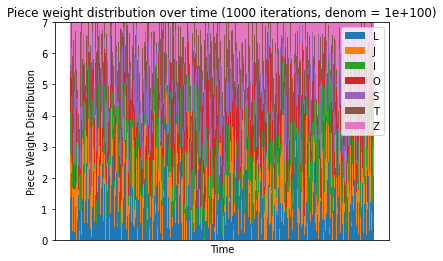```
```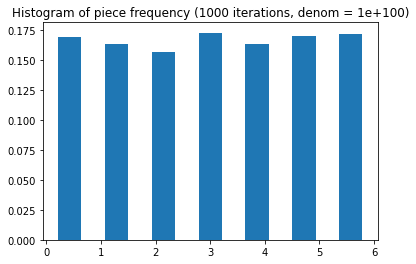```

It looks like this is the limit. At this point, the simulation is basically just setting the weight of the chosen piece to zero every time, and setting the other pieces to make up for it.

This means that it's impossible to get 2 pieces in a row, but it doesn't mean that the piece frequencies are too smoothed out either. Clearly, the denominator of 1.1 was a better balance than this, allowing for consecutive piece-pulls while also preventing droughts.

Is there a better way to evaluate these? We could try minimizing the standard deviation of the piece frequencies, assuming we want to get frequencies to line up.

```def frequency_standard_deviation(pieces):
frequencies = pd.Series(pieces).value_counts() / len(pieces)
return frequencies.std()

def denominator_avg_std(denominator, num_trials, run_length):
total_sd = 0
for _ in range(num_trials):
_, pieces = simulate(run_length, denominator)
total_sd += frequency_standard_deviation(pieces)

DENOMINATORS = np.arange(1.0, 10.0, 0.01)
NUM_TRIALS = 100
RUN_LENGTH = 100

results = pd.DataFrame(DENOMINATORS, columns=['denominator'])

results['avg_std'] = results['denominator'].apply(
lambda denom: denominator_avg_std(denom, NUM_TRIALS, RUN_LENGTH)
)
```
```plt.plot(results['denominator'], results['avg_std'])
plt.xlabel('Denominator')
plt.ylabel('Standard deviation of piece frequency')
plt.xticks(np.arange(1, 10.5, 0.5), rotation='vertical')
plt.title('Standard deviation of piece frequency vs. denominator')
plt.show()
```
```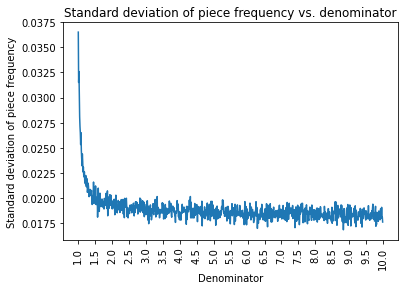```

It looks like this frequency standard deviation metric doesn't really take into account the idea of a "balance" as much as it looks at just minimizing the possibility that frequency could be overly large. This unfortunately doesn't help us much, as we'd like to get to a middle ground between the choosing pieces uniformly and randomly and preventing pieces from being chosen twice in a row.

Something to notice here is that there appears to be a sort of inflection point in between the denominators of 1.0 and 1.5. Let's take a closer look.

```FOCUSED_DENOMINATORS = np.arange(1.0, 1.5, 0.001)

focused_results = pd.DataFrame(FOCUSED_DENOMINATORS, columns=['denominator'])

focused_results['avg_std'] = focused_results['denominator'].apply(
lambda denom: denominator_avg_std(denom, NUM_TRIALS, RUN_LENGTH)
)
```
```plt.scatter(focused_results['denominator'], focused_results['avg_std'], marker='.', s=10)
plt.xlabel('Denominator')
plt.ylabel('Standard deviation of piece frequency')
plt.xticks(np.arange(1, 1.5, 0.1), rotation='vertical')
plt.title('Standard deviation of piece frequency vs. denominator')
plt.show()
```
```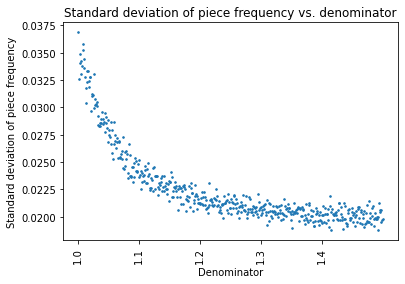```

It looks like the inflection point was around 1.1 all along!

## Conclusions

This randomizer strategy seems okay, as long as a sensible denominator is chosen for the simulation. I wouldn't choose something too large (e.g., anything over 1.5) but would also steer clear of denominators that are too close to 1.0, otherwise the risk of droughts increases.

Here are a couple of sample 100-piece queues, for use with four-tris or similar programs.

```# convert from integers to piece names
def names(pieces):
return ''.join([PIECES[piece] for piece in pieces])
```

Denominator = 1.05

```_, pieces = simulate(100, 1.05)

print(names(pieces))
```
```JLOSZOZTTOTIOJLSISIJTJTJTZZISLLZOTIZSTTOZIZJLLOZISJJIOJLLIOTSOSIJLJJTSTLZSJIJZLIOTZLZZOIZOOTZLTJIISJ

```

Denominator = 1.075

```_, pieces = simulate(100, 1.075)

print(names(pieces))
```
```TSSJSISOOISSOLLOZTSILJTTJOZTSOOLIJJZOZTIOIZJOLSIOIOSLSIJLZLLIJJOLZJJSJJOLSIIOSTZLTLJSJLJILLJTOISISLI

```

Denominator = 1.1

```_, pieces = simulate(100, 1.1)

print(names(pieces))
```
```IZOJZSIIISIISJZLZZTTSLTJSTSJZSZTLJLIJSIOLJOZJOSJZIJSZZSTLTOIITJOLZTILJTOOIJOSLLOSZOTZOZIJTLTSLSIZOOZ

```

Denominator = 1.2

```_, pieces = simulate(100, 1.2)

print(names(pieces))
```
```ILOZTSZOSSZILLTOZTSOJTIIZLZZJTTTSOOISZJLTLTIITOLTSOZSJIOOZIJZLJSSTSLOIJOITTZZLLISLIOSJOJOTOJOTOLTZII

```

Denominator = 1.3

```_, pieces = simulate(100, 1.3)

print(names(pieces))
```
```JJLLSJSTIOJZSOLOILTLZZJZOOLITIZTTLJTSOIJLZOSISZSSTJTLZOIIOTJLZSSJOJZITILJZTTSIZOLOLSZOJSTLSZOLJZOTTO

```

Denominator = 1.4

```_, pieces = simulate(100, 1.4)

print(names(pieces))
```
```OLZTIOLTZOZJJSZIJJZTILOJTZTSLTIJZLSOJSJZSJZTITOSJZISLOTZSLTIZJITZIILTSILZLZLJJITSSJILOSOTSZOIOJSZIJO

```

Denominator = 1.5

```_, pieces = simulate(100, 1.5)

print(names(pieces))
```
```ITZISZOLIILJOJTSJOTOZSZIOILLISSZJSTTLTOOZSIOJLZIILTOTJZLITZSJSSJOJSTZZOLSOZISOOTOSIJTIOTZIITLIJLOZLL

```

Denominator = 2.0

```_, pieces = simulate(100, 2.0)

print(names(pieces))
```
```OZISOLLZITLITSZTOJSOJLZSILSITOTZTJIJJZJSJOZZSTOTLLIJJSITJZOOILIZTOTLOOSJOTOILIJZSISJLLOOZLTZIZJLIOTI

```

Denominator = 5.0

```_, pieces = simulate(100, 5.0)

print(names(pieces))
```
```OLJSIJLOSTOLZJOILZIOTJSOSIZZTJLZIOZSTLSLOJOTLIZTJSIITZTLZOSJIZTISLJOIJTOZOSSJILTILJOZSZZLTOJSLOILIZJ

```